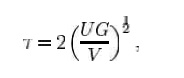Home | | Mechanics of Solids | Beam shear

# Beam shear

Beam shear is defined as the internal shear stress of a beam caused by the shear force applied to the beam.

Beam shear

Beam shear is defined as the internal shear stress of a beam caused by the shear force applied to the beam.where

V = total shear force at the location in question;

Q = statical moment of area;

t = thickness in the mat erial perpendicular to the shear;

I = Moment of Inertia of the entire cross sectional area.

This formula is also known as t he Jourawski formula.

Semi-monocoque shear

Shear stresses within a semi- monocoque structure may be calculated by idealizing the cross-section of the structure into a set of stringers (carrying only axial loads ) and webs (carrying only shear flows). Dividing th e shear flow by the thickness of a given portion of the semi-monocoque structure yields the shear stress. Thus, the maximum shear stre ss will occur either in the web of maximum shear flo w or minimum thickness.

Also constructions in soil can fail due to shear; e.g., the we ight of an earth-filled dam or dike may cause th e subsoil to collapse, like a smalllandslide.

Impact shear

The maximum shear stress crea ted in a solid round bar subject to impact is g iven as the equation:where

U = change in kinetic e nergy;

G = shear modulus;

V = volume of rod;

AndStudy Material, Lecturing Notes, Assignment, Reference, Wiki description explanation, brief detail
Civil : Mechanics Of Solids : Transverse Loading On Beams And Stresses In Beam : Beam shear |